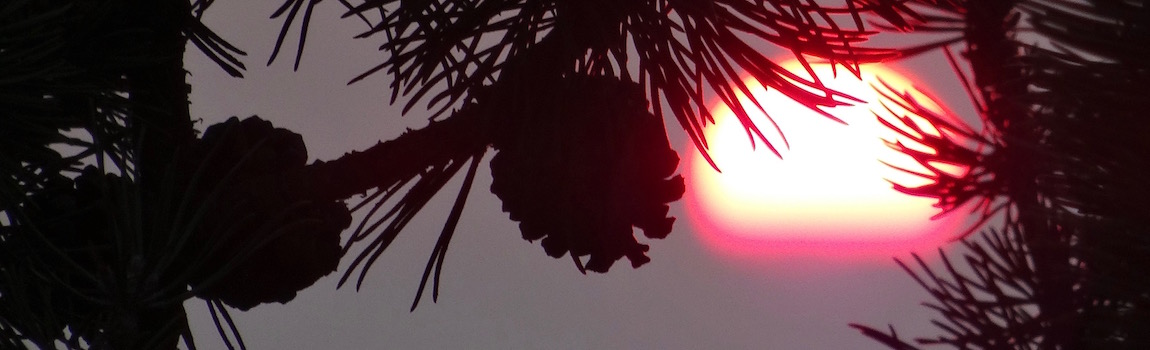Light is refracted and under certain circumstances reflected. Refraction and reflection are one of the most important basics of light.

1 The lost coin

Magic: Why does the coin disapear?

Why is it so difficult to catch a fish with a spear?

How is a rainbow created?

The lost coin in a glas of water is a mystery which can be solved with the laws of optics. Here you will find answers ...

2 refraction of light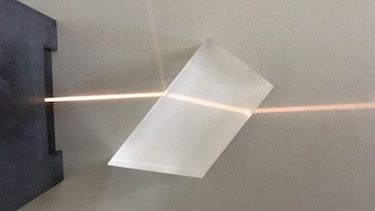If light is hitting a transperant material such as water or glas it is slowed down. If it comes at an angle onto a transparent material it is refracted. In order of the optical denser Material it is refracted toward the lot

Therefore the path of light gets an offset. Since our mind does not know this, the object apears somewhere else. That is why it is difficult to hunt fishes by spear. On the other hand headup-displays use this characteristics to give directions in front of the screen of a car.

3 Snell's Law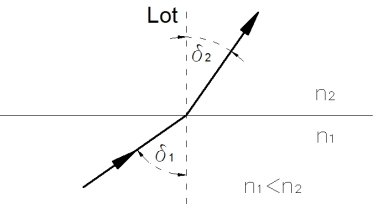The more the light is slowed down in a medium, the higher the refractive index $n$ of the medium. So we can also say: The more the refraction, the higher the refractive index.

With the angle of refraction of a ray the refractive index can be evaluated:

$$n_1 \cdot sin \delta_1 = n_2 \cdot sin \delta_2$$
 Material air H2O glas Si $n$ 1 1.33 1.42 3.2
4 Refractive index and the speed of lightSo we can also calculate the speed of light $c_m$ in a different media.

$$c_m=\frac{c}{n}$$

If light travels from optical denser material (water) into optical less densely material (air) it is refracted away from the lot. If the angle reaches 90 ° it reaches its critical angle angle, meaning all light is reflected and can not get anymore through the transition.

Here one can observe reflection in the aquarium due to the critical angle. That is why fishes inside an aquarium do have a different perspective, than people from outside.

5 Critical angle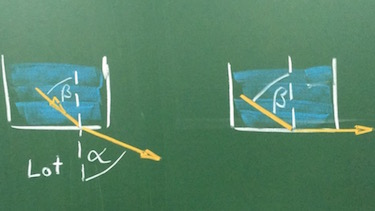The critical angle $\delta_c$ can be easely derived. It occurs if the refracted angle reaches 90 °, thus $\sin \delta_c = 1$:

With Snell's law we get:

$$sin \delta_c = \frac{n_2}{n_1},~ \text{mit}~ n_1 > n_2$$
6 Dispersion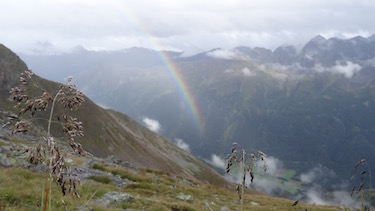The refractiv index of water changes with wavelength. So different colors will be slowed down differently. The color dependent refraction is named dispersion and becomes visible in a rainbow.

 wave­length λ in nm 400 450 500 550 600 650 refrac­tive index n 1.344 1.341 1.336 1.334 1.333 1.332

sources:
K. Johnson et. al., "Advanced Physics for you", Oxford, 2015
Wikipedia, "Electromagnetic Spectrum"
D.K. Lynch and W. Livingston, "Color and light in nature", Cambridge University Press, 2001

#### Experiment 1 Snell's Law | 1580-1626

idea by J. Blum, Christliche Deutsche Schule Chiang Mai

Even named after the Dutch astronomer Willebrord Snellius, Snell's Law was first discribed in 984. To examine refraction you will need a light source with a beam, a prism and a possibility to messure different angles (triangle ruler).1. Take a photo of your experiment.
2. Set the incident ray and measure the refracted angle. Calculate the sinus and the ratio.
 inci­dent angle α 10 ° 20 ° 30 ° 40 ° 50 ° 60 ° 70 ° 80 ° 90 ° sin α refrac­ted angle β sin β sin α/sin βAs a reference you will get one value.

 inci­dent angle α 30 ° refrac­ted angle β 20 sin α/sin β 1.46

#### Question 1 Refraction and critical angle

Construct the ray profile

• Construct the profile of the incident ray. Take into account the critical angle between water and air. Calculate all angles.
• Argue why the coin is lost.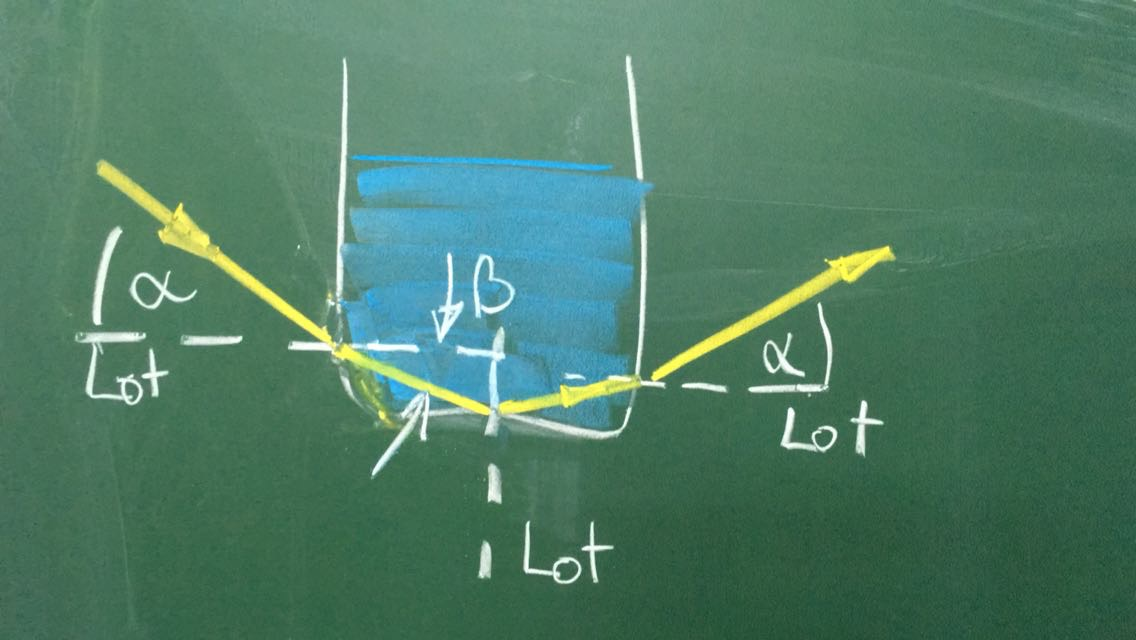#### Question 2 fish in a pond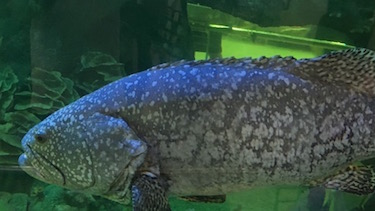Inside a pond is a fish.

1. Determine the viewing area of a fish in a pond if the critical angle is 49 °.
2. Argue how you have to throw a spear in oder to hit the fish in the pond. The fish is 45 ° in front of you.
1.2.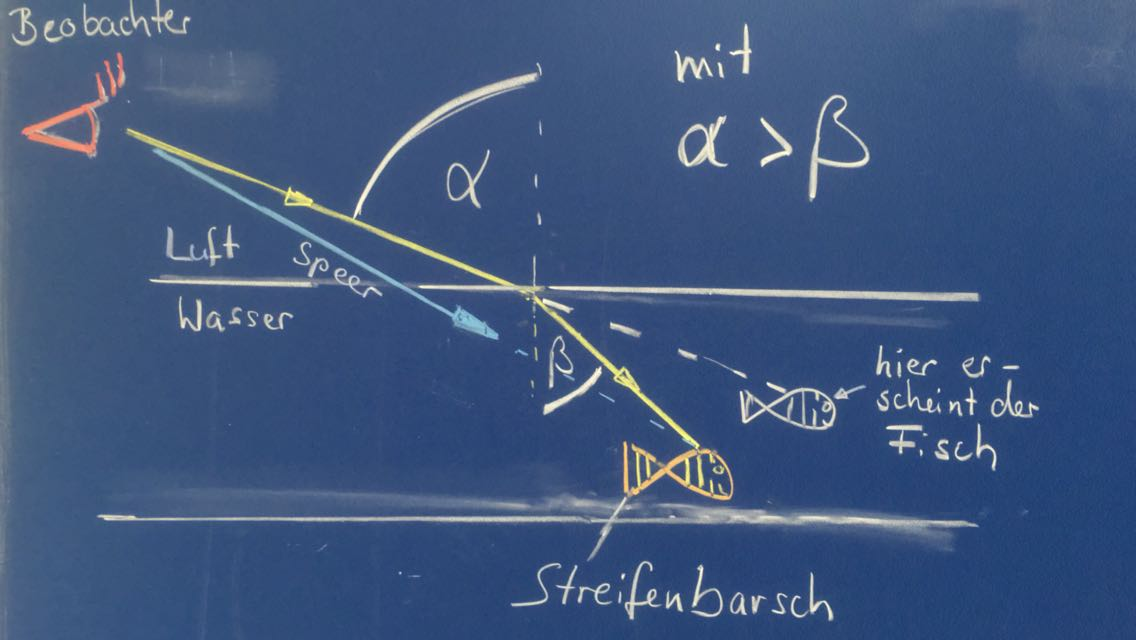### First relax ...

#### Experiment 2 Fermat's principle - Pierre de Fermat 1607-1665Idea: M. Kramer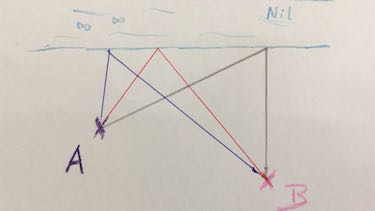Fermat's principle states, that light takes the fastets path. If it is reflected on a mirror, the reflection law states that the incident angle must be similar to the reflected angle of light. But right there the path of light reaches its shortes and fastest way. So Pierre de Fermat had an interessting point of view..

Just take a 10 m rope and imagine the following situation: You are at point A. Before you come to point B you must get water from the riverside of Nil. But you would like to take shortes and thus fastest way.

1. Which way should you take? Get the different opinions of all students.
1. Try different variants and check the length of the rope. Hint: The person in point A holds the rope tight, the person on the river, turns the rope around their leg and the person in point B takes or releases the rope. This is the easiest way to find the shortest way.
2. Now it's becoming more complicated: First you have to go to the forest to make a mug out of leaves. Then go to the river and then to the burning house to clear it. How does the shortest way look like, are there several? If you have found a solution, compare it with the reflection law.
3. Now we leave the stage: Everyone notes the results of Fermat's principle. Perhaps the teacher will reward your great effort with a good grade.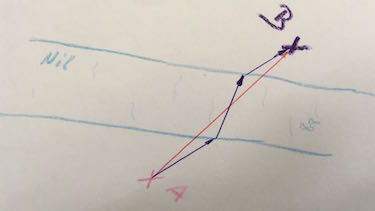Fermat's principle states that light travels the fastest way. Up to now it was even the shortest. No it will be different, since travelling throug water light will be slowed down.

Try to act these situation. Going through water means just having at least half the speed as on lawn.

1. Which way you have to choose in oder to be fastest? Is this way even the shortest?

Here are some statements of students:

1. "Light always takes the shortest way."
2. "The angle of the incident ray is equal to the angle of the reflected ray."
3. "If you panic, you do not think anymore about the shortest way, you just run. Lucky light, just knows the shortest way by itself."
4. "Every path seems to have identical length."

sources:
Interested in more interactie experimente?
M. Kramer, "Physik als Abenteuer", Band 1, Aulis Verlag 2011

#### Question 3 coupling light into an optical fibre

Optical fibres lead light over kilometers since the losses are very small and the speed to transport information is incredible fast. But first you have to get the ray of a laser into the optical fibre. Since the crital angle of this fibre is γ = 76 °, the angle α of the incident ray is restricted.

1. Calculate the refractive index of the cladding $n_{cl}$.
2. Calculate the maximum angle α of the incident ray.
3. Derive a formula for the maxium angle α of the incident ray in depandence of the refractice index $n_{co}$ and $n_{cl}$.1. $n_{co} \cdot \sin(\gamma)=n_{cl} \cdot \sin (90\:°)\: \Rightarrow \:n_{cl}=1,455$
2. $n_{air}\cdot \sin(\alpha)=n_{co} \cdot \sin (90\:°-\gamma)$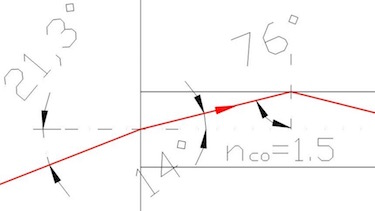3. $\sin(\alpha°)=\frac{n_{co}}{n_{air}} \cdot \sin \left(90\:°- \sin^{-1}\left(\frac{n_{co}}{n_{air}}\right)\right)$

#### Question 4 The rainbow

Little raindrops in the alpes do refract and reflect the light. That is why the sun must be behind you and the rain in front of you, in order to see any rainbow.1. Which color is refracted most, which at least?
2. Which color is slowed down most in water?
3. What is the scientific name for color dependant refraction?
4. Calculate the refracted angle for red and blue light, if the incident ray has an angle of 50 °
5. A prism has a rectangular shape with angles of 60 °. The incident ray has an angle of 50 °. Calculate the refracted angles and draw the rays for red and blue light assuming the prism is made out of water.
6. Name the two colors which are not visible for humans.
1. The blue color is refracted most. That is why blue is visible at the bottom color of the rainbow. Less refraction occurs to red light, since it is at the top of the rainbow.
2. The blue color.
3. The color dependent refraction is named dispersion.
4. Ultraviolet (UV) und Infrarred (IR) light.

### Terms and phrases

 refraction of light light changes its direction in a different medium, since it is slowed down reflection of light light is thrown back in a certain angle refractive index $n$ ratio of speed of light in vacuum and a medium
 speed of light $c$ the speed of light in air or vaccum is $c=300 \cdot 10^6\:m/s$ critical angle the angle when light is not anymore refracted by its transition from a denser medium to a less dense medium dispersion wavelength dependent refraction